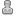# Secure Symmetric Key Exchange

0 Members and 1 Guest are viewing this topic.

####Chaosje##### Secure Symmetric Key Exchange
« on: April 20, 2019, 04:01:02 PM »
Toch geinig wat je kunt bedenken op een blauwe maandag in april 2019http://domero.nl/Secure_Symmetric_Key_Exchange.pdf

Secure Symmetric Key Exchange
2019, Michel SJ Kuipers, Groningen, the Netherlands.
E-mail: chaosje@gmail.com

1. Introduction
Symmetric Key Exchange is a method where one person (classically called Bob) sends a shared key S to another person (classically called Alice) he wishes to use for an encrypted data communication line.
The classical solution takes a function f, which usually is the XOR function with the property:
f(f(A,B),A) = B and f(f(A,B),B) = A
Bob can encode S with a private key B to f(B,S) and send it to Alice.
Alice now encodes f(B,S) with a private key A to f(f(B,S),A) and returns it to Bob
Bob now removes his private key by using f(f(f(B,S),A),B) = f(A,S) and sends it to Alice
Alice removes the private key using f(f(A,S),A) = S and has the shared key.

2. Problem
On an insecure connection, a man-in-the-middle-attack can be performed, by eavesdropping on the communication between Bob and Alice.  A hacker knows f(B,S), f(f(B,S),A) and f(A,S).
By calculation f(f(f(B,S),A),f(B,S)) the hacker determines A. Then by calculating f(f(A,S),A) he can determine S, and listen in on the encrypted connection.

3. Solution
If we can find another function g, where for some or all x, f(x) !=  g(x) but with the same property g(g(A,B),A) = B and g(g(A,B),B) = A, we can randomly choose between f and g, so  a hacker does not know whether to use f or g, making the man-in-the-middle-attack unusable.
We find this function to be the XAND function which has the special property A XAND B = NOT(A XOR B) and A XOR B = NOT(A XAND B). So it’s the inverse of the XOR.
We now can use a beautiful logical property.
Let f be a function, either XOR or XAND.
Let g be a function, either XOR or XAND.
We now have the axiom f(g(f(B,S),A),B) = g(A,S). In our handshake Bob only needs f, and Alice only needs g. Because the other party does not need to know what f or g is, they can keep this a secret, making it impossible for a hacker to determine which is used. The encryption is now finalized by using XOR or XAND randomly on every bit to encode.

4. Implementation
Bob wants to send random key S to Alice.
Bob and Alice create private keys B and A.
Now Bob and Alice create a second private key with the same length as A and B, Q for Bob and R for Alice.
Bob calculates E = B XOR S.
For every bit in E we look at the bit at the same position in Q, if it is a 1 we flip the bit in E, making these bits use the XAND function. Then we send E to Alice.
Alice calculates F = A XOR E, and flips the bits of F if the corresponding bit in R is 1, and sends F to Bob.
Bob now calculates G = F XOR B, and once again flips all corresponding bits in G if the bit in Q is 1, and sends G to Alice.
Alice now calculates T = G XOR A, and flips the corresponding bits in T where the bit in R is 1.
T is now equal to S, and we can set up an encoded communication.
A hacker now can only determine R(B,S) or Q(A,S) but doesn’t know R nor B nor Q nor A so can impossibly determine S.

5. Example
Bob         Alice
Shared Key      10011101 S
Private Key      10110001 B      00111001 A
Private Function Key   00110101 R      11001111 Q

Bob -> Alice: R(B,S)               Alice -> Bob: Q(A,R(B,S)) = Q(A,E)
B 10110001                  A 00111001
S 10011101                  E 00011001
-------------- XOR                  -------------- XOR
X 00101100                  X 00100000
R 00110101                  Q 11001111
-------------- Flip X if R = 1            --------------- Flip X if Q = 1
E 00011001                  F 11101111

Bob -> Alice: R(B,Q(A,R(B,S))) = R(B,F)            Alice: Q(A,R(B,Q(A,R(B,S)))) = Q(A,G)
B 10110001                  A 00111001
F 11101111                  G 01101011
-------------- XOR                  -------------- XOR
X 01011110                  X 01010010
R 00110101                  Q 11001111
-------------- Flip X if R = 1            --------------- Flip X if Q = 1
G 01101011                  T 10011101
S 10011101   Successfully sent.